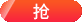C语言四种程序结构

(1) 顺序结构

c = a;

a = b;

b = c;

a = b;

c = a;

b =c;

(2) 分支结构

①if(条件){分支体}

②if(条件){分支1} else {分支2}

1. main()

2. {

3. int a,b,c,d,x,y;

4. printf("Please put the number of a,b&c from the quadratic equation of one variable one by one\n");

5. scanf("%d%d%d",&a,&b,&c);

6. d=b*b-4*a*c;

7. if(d<0)

8. {

9. printf("NO Root!Wrong!\n");

10. }

11. else

12. {

13. y=-b-sqrt(d)/2*a;

14. x=-b+sqrt(d)/2*a;

15. printf("The 1st equation root=%d\nThe 2nd equation root=%d",y,x);

16. }

17. }

③IF嵌套分支语句：

1. if(条件1) {分支1}

2. else if(条件2) {分支2}

3. else if(条件3) {分支3}

4. ……

5. else if(条件n) {分支n}

6. else {分支n+1}

7. FOR嵌套，其语句格式为：

8. for(初值A;范围A;步长A)

9. {

10. for(初值B;范围B;步长B)

11. {

12. 循环体

13. }

14. }

FOR嵌套例子：九九乘法表

1. main()

2. {

3. int a,b,c;

4. for(a=1;a<=9;a++)

5. {

6. for(b=1;b<=a;b++)

7. {

8. c=b*a;

9. printf("%dx%d=%d ",b,a,c);

10. }

11. printf("\n");

12. }

13. }

④switch开关语句

(3)循环结构：

(4)模块化程序结构

C语言的模块化程序结构用函数来实现，即将复杂的C程序分为若干模块，每个模块都编写成一个C函数，然后通过主函数调用函数及函数调用函数来实现一大型问题的C程序编写，因此常说：C程序=主函数+子函数。 因此，对函数的定义、调用、值的返回等中要尤其注重理解和应用，并通过上机调试加以巩固。

相关阅读